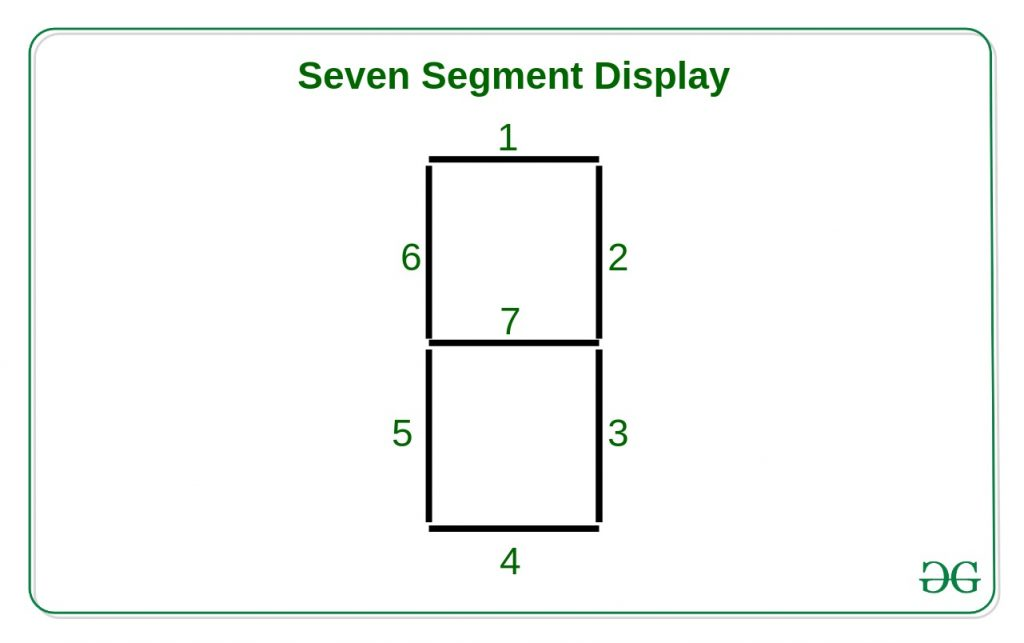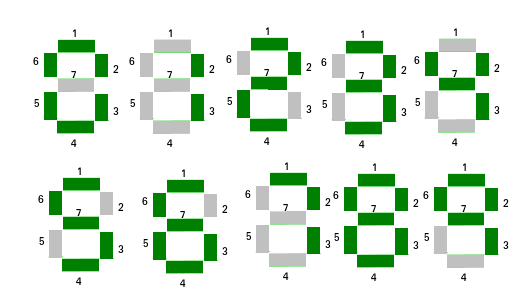# Java Applet | How to display a Digital Clock

In this article, we shall be animating the applet window with a 1-second delay. The idea is to display the system time of every instance.Approach:
Here 6 seven-segment displays are created using the Java Applet library to print the system time in HH:MM:SS format. Each segment of the seven-segment display, numbered as follows can be lit in different combinations to represent the numbers 0-9.

We have 6 seven-segment display for displaying the time in HH:MM:SS pattern. Each segment numbered as the following can be lit in different combinations to represent the numbers 0-9.

We can assume every single segment as 1 bit so (0-9) 10 number can make 10 different combinations.
e.g. if we want to display 0 we should lit-up the segments 0, 1, 2, 3, 4, 5. So this combination will make the number (2^0 | 2^1 | 2^2 | 2^3 | 2^4 | 2^5) = 63.Below is the implementation of the above approach:

 `// Java program to illustrate ` `// digital clock using Applets ` ` `  `import` `java.applet.Applet; ` `import` `java.awt.*; ` `import` `java.util.*; ` ` `  `public` `class` `digitalClock ``extends` `Applet { ` ` `  `    ``@Override` `    ``public` `void` `init() ` `    ``{ ` `        ``// Applet window size ` `        ``this``.setSize(``new` `Dimension(``800``, ``400``)); ` `        ``setBackground(Color.white); ` `        ``new` `Thread() { ` `            ``@Override` `            ``public` `void` `run() ` `            ``{ ` `                ``while` `(``true``) { ` `                    ``repaint(); ` `                    ``delayAnimation(); ` `                ``} ` `            ``} ` `        ``}.start(); ` `    ``} ` ` `  `    ``// Animating the applet ` `    ``private` `void` `delayAnimation() ` `    ``{ ` `        ``try` `{ ` ` `  `            ``// Animation delay is 1000 milliseconds ` `            ``Thread.sleep(``1000``); ` `        ``} ` `        ``catch` `(InterruptedException e) { ` `            ``e.printStackTrace(); ` `        ``} ` `    ``} ` ` `  `    ``// Function that recieves segment combination value ` `    ``// for each digit, position of the display ` `    ``public` `void` `display(``int` `val, ``int` `pos, Graphics g) ` `    ``{ ` ` `  `        ``// lit-up the i-th segment ` ` `  `        ``// 0-th segment ` `        ``if` `((val & ``1``) != ``0``) ` `            ``g.fillRect(pos, ``150``, ``5``, ``50``); ` ` `  `        ``// 1-st segment ` `        ``if` `((val & ``2``) != ``0``) ` `            ``g.fillRect(pos, ``145``, ``50``, ``5``); ` ` `  `        ``// 2-nd segment ` `        ``if` `((val & ``4``) != ``0``) ` `            ``g.fillRect(pos + ``45``, ``150``, ``5``, ``50``); ` ` `  `        ``// 3-rd segment ` `        ``if` `((val & ``8``) != ``0``) ` `            ``g.fillRect(pos + ``45``, ``200``, ``5``, ``50``); ` ` `  `        ``// 4-th segment ` `        ``if` `((val & ``16``) != ``0``) ` `            ``g.fillRect(pos, ``250``, ``50``, ``5``); ` ` `  `        ``// 5-th segment ` `        ``if` `((val & ``32``) != ``0``) ` `            ``g.fillRect(pos, ``200``, ``5``, ``50``); ` ` `  `        ``// 6-th segment ` `        ``if` `((val & ``64``) != ``0``) ` `            ``g.fillRect(pos + ``5``, ``200``, ``40``, ``5``); ` `    ``} ` ` `  `    ``// Paint the applet ` `    ``@Override` `    ``public` `void` `paint(Graphics g) ` `    ``{ ` `        ``// Combination values of different digits ` `        ``int``[] digits ` `            ``= ``new` `int``[] { ``63``, ``12``, ``118``, ` `                          ``94``, ``77``, ``91``, ` `                          ``123``, ``14``, ``127``, ` `                          ``95` `}; ` ` `  `        ``// Get the system time ` `        ``Calendar time = Calendar.getInstance(); ` ` `  `        ``int` `hour = time.get(Calendar.HOUR_OF_DAY); ` `        ``int` `minute = time.get(Calendar.MINUTE); ` `        ``int` `second = time.get(Calendar.SECOND); ` ` `  `        ``// Deciding AM/PM ` `        ``int` `am = ``1``; ` `        ``if` `(hour > ``12``) { ` `            ``hour -= ``12``; ` `            ``am = ``0``; ` `        ``} ` ` `  `        ``// Display hour ` ` `  `        ``// tens digit ` `        ``display(digits[hour / ``10``], ``150``, g); ` `        ``// units digit ` `        ``display(digits[hour % ``10``], ``225``, g); ` ` `  `        ``// Display minute ` ` `  `        ``// tens digit ` `        ``display(digits[minute / ``10``], ``325``, g); ` `        ``// units digit ` `        ``display(digits[minute % ``10``], ``400``, g); ` ` `  `        ``// Display second ` ` `  `        ``// tens digit ` `        ``display(digits[second / ``10``], ``500``, g); ` `        ``// units digit ` `        ``display(digits[second % ``10``], ``575``, g); ` ` `  `        ``// Display AM/PM ` `        ``if` `(am == ``1``) ` `            ``g.drawString(``"am"``, ``650``, ``250``); ` `        ``else` `            ``g.drawString(``"pm"``, ``650``, ``250``); ` ` `  `        ``// Display ratio signs ` `        ``g.fillRect(``300``, ``175``, ``5``, ``5``); ` `        ``g.fillRect(``300``, ``225``, ``5``, ``5``); ` ` `  `        ``g.fillRect(``475``, ``175``, ``5``, ``5``); ` `        ``g.fillRect(``475``, ``225``, ``5``, ``5``); ` `    ``} ` `} `

Output:

Attention reader! Don’t stop learning now. Get hold of all the important DSA concepts with the DSA Self Paced Course at a student-friendly price and become industry ready.

My Personal Notes arrow_drop_upCheck out this Author's contributed articles.

If you like GeeksforGeeks and would like to contribute, you can also write an article using contribute.geeksforgeeks.org or mail your article to contribute@geeksforgeeks.org. See your article appearing on the GeeksforGeeks main page and help other Geeks.

Please Improve this article if you find anything incorrect by clicking on the "Improve Article" button below.

Article Tags :
Practice Tags :

Be the First to upvote.

Please write to us at contribute@geeksforgeeks.org to report any issue with the above content.